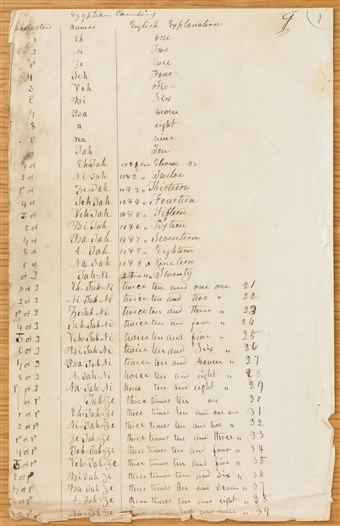# Egyptian Counting, circa Early July–circa November 1835

• Source Note
• Historical Introduction
Page 1Egyptian Counting
<​G​>
 Characters names English Explanation ✦ Eh one ✦ Ni Two ✦ Ze Three ✦ Teh Four ✦ Veh Five ✦ Psi Six ✦ Psa Seven ✦ a eight ✦ na nine ✦ Tah Ten ✦ ✦ Eh<​=​>Tah 10 & 2 1 are Eleven or ✦ ✦ Ni=Tah 10 & 2 " Twelve ✦ ✦ Ze=Tah 10 & 3 " Thirteen ✦ ✦ Teh Tah 10 & 4 " Fourteen ✦ ✦ Veh<​=​>Tah 10 & 5 " Fifteen ✦ ✦ Psi Tah 10 & 6 " Sixteen ✦ ✦ Psa=Tah 10 & 7 " Seventeen ✦ ✦ A=Tah 10 & 8 " Eighteen ✦ ✦ Na=Tah 10 & 9 " Nineteen ✦ ✦ Tah=Ni 10 & 2 Tmes 10 " Twenty ✦ ✦ ✦ Eh=Tah=Ni twice ten and one are 21 ✦ ✦ ✦ Ni=Tah Ni twice ten and two " 22 ✦ ✦ ✦ Ze=Tah Ni twice ten and three " 231TEXT: Possibly “22 23”. ✦ ✦ ✦ Teh=Tah Ni twice ten and four " 24 ✦ ✦ ✦ Veh=Tah Ni twice ten and five " 25 ✦ ✦ ✦ Psi Tah Ni twice ten and Six " 26 ✦ ✦ ✦ Psa Tah Ni twice ten and Seven " 27 ✦ ✦ ✦ A=Tah=Ni twice ten and eight " 28 ✦ ✦ ✦ ✦ ✦ Na=Tah=Ni twice ten and eight " 29 ✦ ✦ Tah=Ze three times ten are 30 ✦ ✦ ✦ Eh=Tah=Ze three times ten and one are 31 ✦ ✦ ✦ Ni=Tah=Ze three times ten and two " 32 ✦ ✦ ✦ Ze=Tah=Ze three times ten and three " 33 ✦ ✦ ✦ Teh=Tah=Ze three times ten and four " 34 ✦ ✦ ✦ ✦2TEXT: The canceled character is possibly the “teh” character associated with and shaped like “4”. Veh=Tah=Ze three times ten and five " 35 ✦ ✦ ✦ Psi Tah Ze three times ten and six " 36 ✦ ✦ ✦ Psa Tah Ze three times ten and seven " 37 ✦ ✦ ✦ A=Tah=Ze three times ten and eight " 38 ✦ ✦ ✦ Na=Tah=Ze [t]hree3TEXT: Wear at the bottom of this page obscures this word.   times ten and nine " 39
[p. 1]
Egyptian Counting
G
 Characters names English Explanation ✦ Eh one ✦ Ni Two ✦ Ze Three ✦ Teh Four ✦ Veh Five ✦ Psi Six ✦ Psa Seven ✦ a eight ✦ na nine ✦ Tah Ten ✦ ✦ Eh=Tah 10 & 1 are Eleven or ✦ ✦ Ni=Tah 10 & 2 " Twelve ✦ ✦ Ze=Tah 10 & 3 " Thirteen ✦ ✦ Teh Tah 10 & 4 " Fourteen ✦ ✦ Veh=Tah 10 & 5 " Fifteen ✦ ✦ Psi Tah 10 & 6 " Sixteen ✦ ✦ Psa=Tah 10 & 7 " Seventeen ✦ ✦ A=Tah 10 & 8 " Eighteen ✦ ✦ Na=Tah 10 & 9 " Nineteen ✦ ✦ Tah=Ni 2 Tmes 10 " Twenty ✦ ✦ ✦ Eh=Tah=Ni twice ten and one are 21 ✦ ✦ ✦ Ni=Tah Ni twice ten and two " 22 ✦ ✦ ✦ Ze=Tah Ni twice ten and three " 23 ✦ ✦ ✦ Teh=Tah Ni twice ten and four " 24 ✦ ✦ ✦ Veh=Tah Ni twice ten and five " 25 ✦ ✦ ✦ Psi Tah Ni twice ten and Six " 26 ✦ ✦ ✦ Psa Tah Ni twice ten and Seven " 27 ✦ ✦ ✦ A=Tah=Ni twice ten and eight " 28 ✦ ✦ ✦ Na=Tah=Ni twice ten and eight " 29 ✦ ✦ Tah=Ze three times ten are 30 ✦ ✦ ✦ Eh=Tah=Ze three times ten and one are 31 ✦ ✦ ✦ Ni=Tah=Ze three times ten and two " 32 ✦ ✦ ✦ Ze=Tah=Ze three times ten and three " 33 ✦ ✦ ✦ Teh=Tah=Ze three times ten and four " 34 ✦ ✦ ✦ Veh=Tah=Ze three times ten and five " 35 ✦ ✦ ✦ Psi Tah Ze three times ten and six " 36 ✦ ✦ ✦ Psa Tah Ze three times ten and seven " 37 ✦ ✦ ✦ A=Tah=Ze three times ten and eight " 38 ✦ ✦ ✦ Na=Tah=Ze three times ten and nine " 39
[p. 1]
Page 1